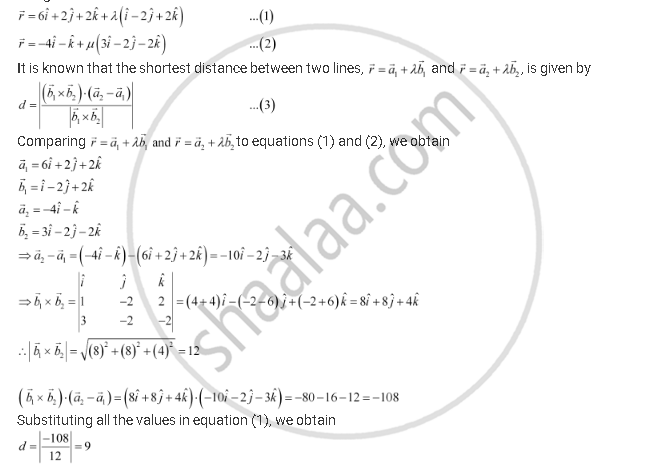Share

# Find the Shortest Distance Between Lines Vecr = 6hati + 2hatj + 2hatk + Lambda(Hati - 2hatj + 2hatk) and Vecr =-4hati - Hatk + Mu(3hati - 2hatj - 2hatk) - CBSE (Science) Class 12 - Mathematics

ConceptShortest Distance Between Two Lines

#### Question

Find the shortest distance between lines vecr = 6hati + 2hatj + 2hatk + lambda(hati - 2hatj + 2hatk) and vecr =-4hati - hatk + mu(3hati - 2hatj - 2hatk)

#### Solution

The given lines areTherefore, the shortest distance between the two given lines is 9 units.

Is there an error in this question or solution?

#### APPEARS IN

NCERT Solution for Mathematics Textbook for Class 12 (2018 to Current)
Chapter 11: Three Dimensional Geometry
Q: 9 | Page no. 498

#### Video TutorialsVIEW ALL 

Solution Find the Shortest Distance Between Lines Vecr = 6hati + 2hatj + 2hatk + Lambda(Hati - 2hatj + 2hatk) and Vecr =-4hati - Hatk + Mu(3hati - 2hatj - 2hatk) Concept: Shortest Distance Between Two Lines.
S